GeoA - Enrichment 3
Tell me that you did this when you are finished
As a lifeguard, you earn \$6 per day plus \$2.50 per hour. How many hours do you need to work to make \$16 in one day? *
Evaluate if a = 12, b = 9, and c = 4. *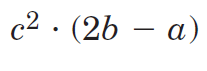Segment EP is the bisector of <GEF. Find x. *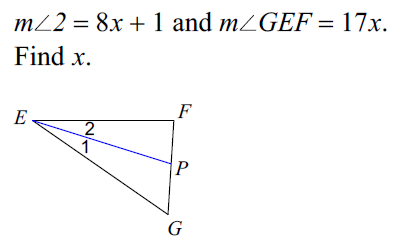period *
Last Name *
Solve for y *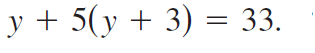Solve for a. BD is an angle bisector *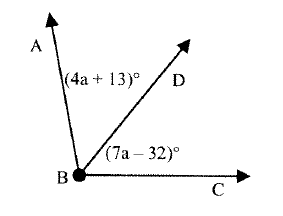Solve for n *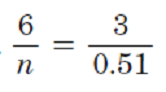Find the slope of a line that passes through the points (-3, 2) and (1, 5) *
Write this line in slope-intercept form *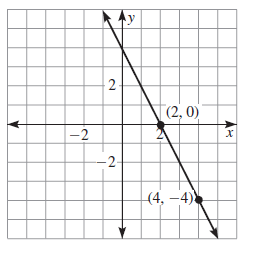Find the slope of the equation x + 3y - 6 = 0 *
solve *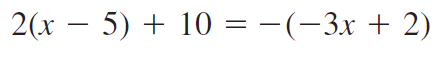Solve for p *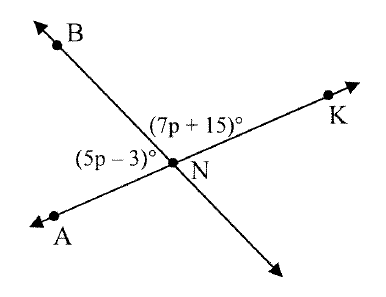B is the midpoint of segment AC. If BC= 5x - 9 and AB = 2x + 21. Find x. *
Never submit passwords through Google Forms.
This content is neither created nor endorsed by Google. Report Abuse - Terms of Service - Privacy Policy